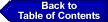### Benchmarks for Science Literacy: Chapter 15THE RESEARCH BASE

9 THE MATHEMATICAL WORLD

9D) UNCERTAINTY

Students' conceptions about uncertainty and students' probabilistic reasoning have been extensively researched, and there are several literature reviews on the topic (Garfield & Ahlgren, 1988; Hawkins & Kapadia, 1984; Shaughnessy, 1992). The research on summarizing data, which focuses on students' understanding of different measures of central tendency and dispersion, is less extensive.

Probability. Research presents somewhat contradictory results on elementary children's understanding of probability. Piagetian research says lower elementary children have no conception of probability (Piaget & Inhelder, 1975; Shayer & Adey, 1981), but other studies indicate that even lower elementary-school children have probabilistic intuitions upon which probability instruction can build. Falk et al. (1980) presented elementary-school students with two sets, each containing blue and yellow elements. Each time, one color was pointed out as the payoff color. The students had to choose the set from which they would draw at random a "payoff element" to be rewarded. From the age of six, children began to select the more probable set systematically. The ability to choose correctly precedes the ability to explain these choices.

Upper elementary students can give correct examples for certain, possible, and impossible events, but cannot calculate the probability of independent and dependent events even after instruction on the procedure (Fischbein & Gazit, 1984). That is partly because students at this age tend to create "part to part" rather than "part to whole" comparisons (e.g., 9 men and 11 women rather than 15% of men and 10% of women). By the end of 8th grade, students can use ratios to calculate probabilities in independent events, after adequate instruction (Fischbein & Gazit, 1984).

Upper elementary students begin to understand that there is an increase in regularity of a sample distribution with an increase in the sample size, but they can apply this idea only to relatively small numbers. It is postulated that to deal with large numbers, children must first cope with notions of ratio and proportion and that their failure to understand these notions creates "a law of small large numbers" (Bliss, 1978).

Extensive research points to several misconceptions about probabilistic reasoning that are similar at all age levels and are found even among experienced researchers (Kahneman, Slovic, & Tversky, 1982; Shaughnessy, 1992). One common misconception is the idea of representativeness, according to which an event is believed to be probable to the extent that it is "typical." For example, many people believe that after a run of heads in coin tossing, tails should be more likely to come up. Another common error is estimating the likelihood of events based on how easily instances of it can be brought to mind.

Summarizing data. The concept of the mean is quite difficult for students of all ages to understand even after several years of formal instruction. Several difficulties have been documented in the literature: Students of all ages can talk about the algorithm for computing the mean and relate it to limited contexts, but cannot use it meaningfully in problems (Mokros & Russell, 1992; Pollatsek, Lima, & Well, 1981); upper elementary- and middle-school students believe that the mean of a particular data set is not one precise numerical value but an approximation that can have one of several values (Mokros & Russell, 1992); some middle-school students cannot use the mean to compare two different-sized sets of data (Gal et al., 1990); high-school students may believe the mean is the usual or typical value (Garfield & Ahlgren, 1988); students (or adults) may think that the sum of the data values below the mean is equivalent to the sum above the mean (rather than that the total of the deviations below the mean is equal to the total above) (Mokros & Russell, 1992).

Research suggests that a good notion of representa-tiveness may be a prerequisite to grasping the defin-itions for measures of location like mean, median, or mode. Students can acquire notions of representa-tiveness after they start seeing data sets as entities to be described and summarized rather than as "unconnected" individual values. This occurs typically around 4th grade (Mokros & Russell, 1992).

Research suggests students should be introduced first to location measures that connect with their emerging concept of the "middle," such as the median, and later in the middle-school grades, to the mean. Premature introduction of the algorithm for computing the mean divorced from a meaningful context may block students from understanding what averages are for (Mokros & Russell, 1992; Pollatsek et al., 1981).